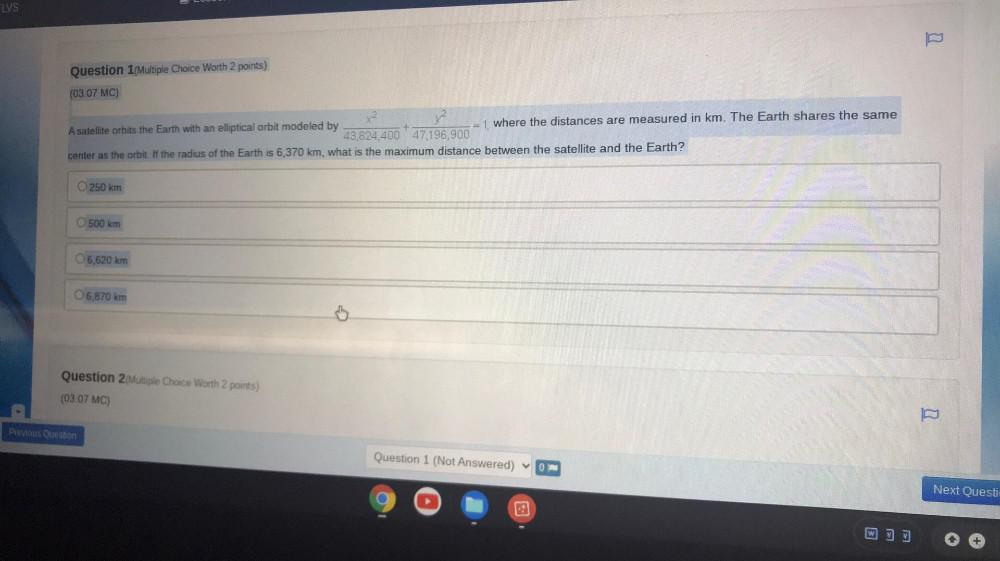Question:

# ELVS Question 1/Multiple Choice Worth 2 points) (03.07 MC) A satellite orbits the Earth with an elliptical orbit modeled by = 1,ELVS Question 1/Multiple Choice Worth 2 points) (03.07 MC) A satellite orbits the Earth with an elliptical orbit modeled by = 1, where the distances are measured in km. The Earth shares the same 43,824,400 47,196,900 center as the orbit. If the radius of the Earth is 6,370 km, what is the maximum distance between the satellite and the Earth? 250 km 500 km 6,620 km O 6,870 km Question 2 Multiple Choice Worth 2 points) (0307 MC) Previous Question Question 1 (Not Answered) ON Next Questi w 13